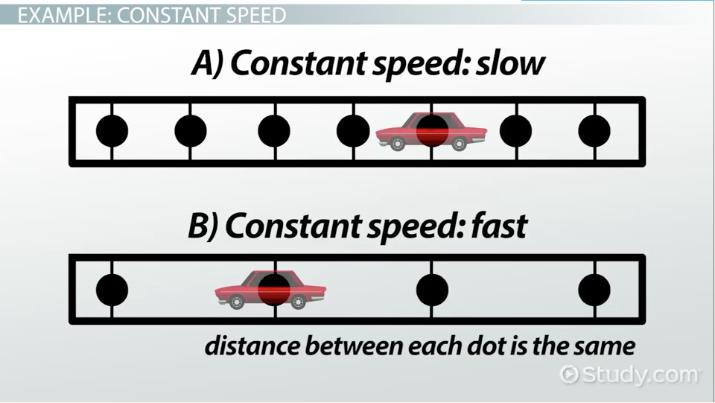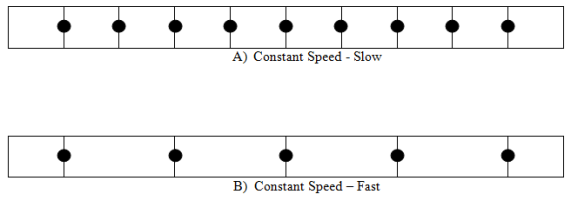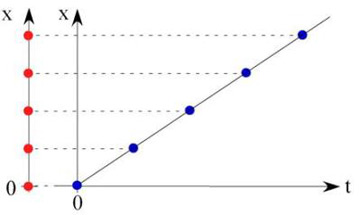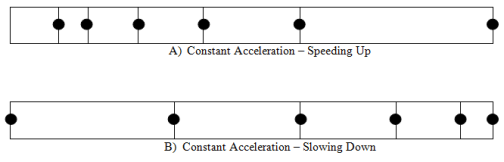# Constant Motion in Physics: Definition & Overview

Lesson Transcript
Instructor: Richard Cardenas

Richard Cardenas has taught Physics for 15 years. He has a Ph.D. in Physics with a focus on Biological Physics.

In this lesson you will learn about the different kinds of constant motion in physics. There are two types of constant motion relevant to physics: constant speed and constant acceleration. In this lesson you will learn the definition of both motions and how they are graphically illustrated.

## Definition of Constant Motion

Constant motion refers to any type of motion when either the distance traveled by the object is the same for each second, or the speed of the object changes by the same amount each second.

Imagine tapping your hand on the table once every half a second, and what that would sound like. Now think about taking one step every half a second. If you methodically take one step every half second, you are moving with a constant speed, one type of constant motion. Constant speed means that you are moving the same distance every second, so your speed does not increase at all.

Now imagine tapping the table once during the first second, twice during the next second, three times during the third second, etc. Now think about taking one step during the first second, two steps in the next second, and three steps during the third second, etc. This process describes another type of constant motion: constant acceleration. In constant acceleration, your speed increases by the same amount every second. So if you have 1 m/s (or meters per second) during the first second, and 2 m/s during the next second (an increase of 1 m/s), then you must travel 3 m/s, 4 m/s, and 5 m/s during the third, fourth, and fifth seconds, etc. Your constant acceleration is 1 m/s/s (meters per second per second or meters per second squared).An error occurred trying to load this video.

Try refreshing the page, or contact customer support.

Coming up next: Convection in Science: Definition, Equation & Examples

### You're on a roll. Keep up the good work!

Replay
Your next lesson will play in 10 seconds
• 0:00 Definition of Constant Motion
• 1:25 Example: Constant Speed
• 2:55 Example: Constant Acceleration
• 4:20 Lesson Summary
Save Save

Want to watch this again later?

Timeline
Autoplay
Autoplay
Speed Speed

## Example: Constant Speed

Now let's discuss an example of constant motion. Let's say you are driving interstate I-10 from San Antonio to El Paso, Texas. Most of the sections of this path are fairly flat and straight. You reach a cruising speed of 75 mph when you decide to engage your cruise control. The cruise control system keeps the vehicle speed at 75 mph until you hit the brakes or accelerate. While you are using the cruise control, you are moving with constant speed. This table illustrates the distance traveled during a four-hour trip on cruise control.

Time Distance
1 hour 75 miles
2 hours 150 miles
3 hours 225 miles
4 hours 300 miles

Based on the table, you traveled 75 miles each hour while you were on cruise control. That is a total of 300 miles for a four-hour trip on cruise control. This is an example of what constant speed data would look like.These figures illustrate constant speed using dots. Each dot shows the location of the object for each one second interval. Figure A represents a slow-moving object since the dots are closer together. Figure B represents a fast-moving object since the dots are farther apart each second. Note that the distance between each dot is the same in each figure. Therefore, the object represented by the dot is moving with constant speed in both cases. These figures make up what we call a motion diagram.

The position versus time graph below illustrates constant speed in a different way. During each second, the object moves the same distance.## Constant AccelerationTo unlock this lesson you must be a Study.com Member.

### Register to view this lesson

Are you a student or a teacher?